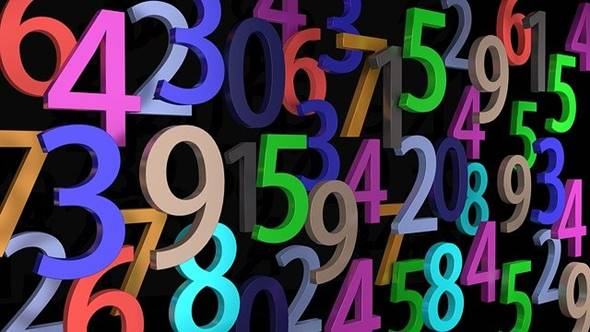# What are Composite Numbers? How to Identify Prime and Composite NumbersIf one is good at the crunching of numbers, then mathematics is no longer a riddle. A basic understanding of this subject can be established by getting acquainted with the types of numbers, especially prime and composite numbers, as students often tend to get confused with them.

Mathematics is a subject that lets us identify trends around patterns and how to use these trends in making new theorems. In offering insight or forecasts about existence in nature too, mathematics serves a vital role with its reasoning and logic.

Mathematics is generated by counting, estimation, measuring, with numbers forming the basis of all the logic; hence numbers play a crucial role in mathematics. Numbers assist us in calculating and weighing quantities; hence they form an essential part of learning the foundation of math. These numbers are categorized as natural, real, integer, rational, irrational, complex etc. Prime and composite numbers, if properly explained, the kids pick them up very well.

For additional help apart from their regular curriculum, they can visit the Cuemath website to find some helpful number worksheets for practice. 2nd-grade math worksheets are also available for younger kids in a fun and interactive manner to understand the concepts of prime and composite numbers.

These worksheets are easy to download and provide enough practice questions to cover the number topic well. Expert maths teachers at cuemath are also available, which can tutor the child online at their homes’ comfort. One can easily book a free trial class to see the teaching methodology that they follow.

The prime numbers are those natural numbers that are only divisible by themselves and by number one. In other words, their factors will be one and the number itself, making it a total of two factors. While if a number can be formed by multiplying two or more numbers, then that is a composite number.

So, there are more than two factors in composite numbers, or we can say a minimum of three factors. One point to remember is that the number 1 is neither prime nor composite. And an easy thing to remember is that the numbers which are not composite numbers can be classified as prime numbers. Let us see how to identify prime and composite numbers.

We use a method called factorization. If we see number 6 and consider its factors, they will be 2 and 3. On multiplying 2 and 3, we will get 6; hence 6 is a composite number and not a prime number. On the other hand, let us consider the number 5; what numbers, when multiplied, gives 5? We can only think of 1 and 5.

Remember, 1 is neither prime nor composite. Thus, 5 is a prime number since it can be divided only by itself. When the only two factors of any number are 1 and the number itself, it is a prime number. In order to find out if a number is a prime or a composite, a divisibility test using the numbers 2, 3, 5, 7, 11, and 13 should be carried out.

If a number is divisible by one of these, that means it’s composite, and no need for further tests. When the factorization process is mastered, the older kids who are allowed to use calculators for complex calculations can find prime or composite by typing in the number and start dividing by 2, then 3 and so on. If the quotient is a whole number, then that means the number is composite and not prime.

Thus, 5 is a prime number since it can be divided only by itself. When the only two factors of any number are 1 and the number itself, it is a prime number. In order to find out if a number is a prime or a composite, a divisibility test using the numbers 2, 3, 5, 7, 11, and 13 should be carried out. If a number is divisible by one of these, that means it’s composite, and no need for further tests. When the factorization process is mastered, the older kids who are allowed to use calculators for complex calculations can find prime or composite by typing in the number and start dividing by 2, then 3 and so on. If the quotient is a whole number, then that means the number is composite and not prime.

This site uses Akismet to reduce spam. Learn how your comment data is processed.